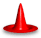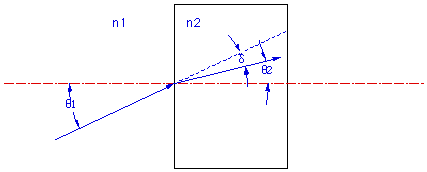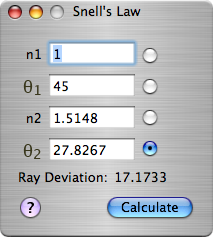OpticsCalc: Snell's LawDescriptionThe Snell's Law page computes the refraction at the boudary between two materials of different indices n1 and n2. The incident ray in material 1 is incident on the interface at angle θ1 with respect to the surface normal. The ray then refracts at the surface and enters material 2 at an angle of θ2 relative to the optical axis. The ray is deviated by a total angle shown as δ in the figure.

n1sin(θ1) = n2sin(θ2)This page allows you to enter any 3 of the 4 values and compute the fourth. This is a manual calculation page so you must fill in the known quantities, select the value you want to calculate by pressing the appropriate radio button and then press Calculate. Note that the ray deviation is computed automatically.A Note On UnitsAll angles are in degrees.